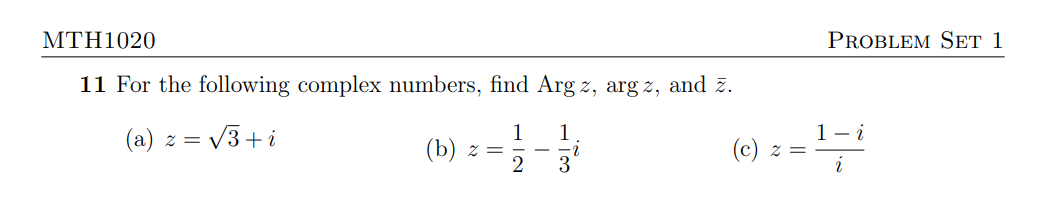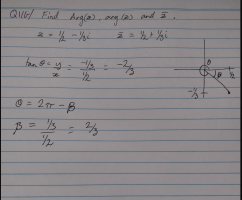# Argument of a complex number that isn't a standard angle

#### Four Muffins

##### New member
Hello. I'm not allowed to use a calculator to solve this, but I'm unable to use either of the methods covered in the unit to solve this problem.

The methods are using arctan rise over run, or to use a 'helper angle', but with either one I end up at the same spot. It is questions b I'm stuck on. Is this supposed to be a trick question that is unsolvable, or would I just leave the argument as arctan -2/3? I do notice that arctan 2/3 is very close to root3/3, but I don't understand if that is a coincidence or not.#### pka

##### Elite Member
Hello. I'm not allowed to use a calculator to solve this, but I'm unable to use either of the methods covered in the unit to solve this problem.

The methods are using arctan rise over run, or to use a 'helper angle', but with either one I end up at the same spot. It is questions b I'm stuck on. Is this supposed to be a trick question that is unsolvable, or would I just leave the argument as arctan -2/3? I do notice that arctan 2/3 is very close to root3/3, but I don't understand if that is a coincidence or not.

View attachment 33587
We want to find the argument of
We want to find the argument of [imath]z=x+y\bf i[/imath]
If [imath]x\cdot y=0[/imath] then you should know that the argument is [imath]\pm\dfrac{\pi}{2},\;0\text{ or }\pi[/imath]
Thus if [imath]x\cdot y\ne 0~\&~x>0 [/imath] then [imath]\arg(z)=\arctan\left(\dfrac{y}{x}\right)[/imath]
If [imath]x<0~\&~y>0[/imath]then [imath]\arg(z)=\pi+\arctan\left(\dfrac{y}{x}\right)[/imath]
If [imath]x<0~\&~y<0[/imath] then [imath]\arg(z)=-\pi+\arctan\left(\dfrac{y}{x}\right)[/imath]
[imath][/imath][imath][/imath][imath][/imath]

Last edited:
•topsquark

#### Dr.Peterson

##### Elite Member
Hello. I'm not allowed to use a calculator to solve this, but I'm unable to use either of the methods covered in the unit to solve this problem.

The methods are using arctan rise over run, or to use a 'helper angle', but with either one I end up at the same spot. It is questions b I'm stuck on. Is this supposed to be a trick question that is unsolvable, or would I just leave the argument as arctan -2/3? I do notice that arctan 2/3 is very close to root3/3, but I don't understand if that is a coincidence or not.

View attachment 33587
View attachment 33588
It sounds like you're just unwilling to actually use the calculator, though you are allowed to.

The answer is, in fact, [imath]\arctan\left(-\frac{2}{3}\right)[/imath] -- unless perhaps you are given a different range to use for the argument. So put that into your calculator, and the number you get is your answer.

Or did you do that, and something more, but didn't attach that part? That may help us better understand your thinking.

I see that the value is [imath]-0.5880026...[/imath], which is indeed somewhat close to [imath]\frac{\sqrt{3}}{3} = 0.57735...[/imath], but that is entirely a coincidence, since angles in radians generally have nothing to do with radicals. (You are probably thinking of the fact that the tangent, not the inverse tangent, of some special angles can be exactly represented by a radical.) If this were a special angle, then the answer would be some nice multiple of [imath]\pi[/imath], not of a radical.

Another possible issue would be the meaning of Arg as opposed to arg; hopefully when you show all your work it will help us be sure what conventions are followed in your class (which do vary a little). In particular, what interval are you required to use for the principal value?

•topsquark

#### Four Muffins

##### New member
Thank you, Dr.Peterson. We were told in our first class that 'we're grown ups now, we don't use calculators'. They're forbidden in the exam as well, so I guess they want us to get used to not relying on them. But we were also told that we would not be given angles that are not the special angles. I'd just ask the lecturer to clarify, but the assignment is due Monday and they don't work weekendsThe conventions we use are Arg (z) = Theta + 2kpi on the interval (-pi, pi], and arg (z) = Theta, counted counter-clockwise.

I did try putting arctan -2/3 into the calculator, which made me pretty sure I either couldn't solve it, or was making an error earlier in my working (I'm rather prone to those...) Since you got the same answer, I suspect that I'm expected to use arctan -2/3 as the argument. It is also nice to know that rounding to special angles is not a thing, haha

#### Dr.Peterson

##### Elite Member
Thank you, Dr.Peterson. We were told in our first class that 'we're grown ups now, we don't use calculators'. They're forbidden in the exam as well, so I guess they want us to get used to not relying on them. But we were also told that we would not be given angles that are not the special angles. I'd just ask the lecturer to clarify, but the assignment is due Monday and they don't work weekendsActually, I missed the word "not" in what you said! Sorry about that.

Yes, they are being inconsistent. Presumably what is true of exams is not true of all homework.

But in fact, adults use calculators, because in real life you don't get nice answers. Ideally, you should be allowed to use calculators for some problems, but not others.

The conventions we use are Arg (z) = Theta + 2kpi on the interval (-pi, pi], and arg (z) = Theta, counted counter-clockwise.
Interesting. Some sources, such as this in Wikipedia, make Arg the principal angle, and arc the general case! But you have the interval they say is common.
I did try putting arctan -2/3 into the calculator, which made me pretty sure I either couldn't solve it, or was making an error earlier in my working (I'm rather prone to those...) Since you got the same answer, I suspect that I'm expected to use arctan -2/3 as the argument. It is also nice to know that rounding to special angles is not a thing, haha
Yes, if they were to give you this on a no-calculator exam, you would just leave it as the exact value in the form arctan([imath]-\frac{2}{3})[/imath].

#### Four Muffins

##### New member
I mixed up Arg and arg, because of course I did, even though I was explicitly told not to. I read arg (z) = Arg (z) +2kpi in a section about ambiguity and and misinterpreted it, haha.

Thanks for your help.#### Dr.Peterson

##### Elite Member
Actually, I missed the word "not" in what you said! Sorry about that.

Yes, they are being inconsistent. Presumably what is true of exams is not true of all homework.

But in fact, adults use calculators, because in real life you don't get nice answers. Ideally, you should be allowed to use calculators for some problems, but not others.

Interesting. Some sources, such as this in Wikipedia, make Arg the principal angle, and arc the general case! But you have the interval they say is common.

Yes, if they were to give you this on a no-calculator exam, you would just leave it as the exact value in the form [imath]\frac{\sqrt{3}}{3}[/imath].
I'm failing to check what I type! I pasted in the wrong expression at the end there; I hope it was clear I meant [imath]\arctan\left(-\frac{2}{3}\right)[/imath]............................fixed

And about Arg vs arg, I hope you noticed that Wikipedia said that both that and the interval vary:

Some authors define the range of the principal value as being in the closed-open interval [0, 2π).​
Note that notation varies, so arg and Arg may be interchanged in different texts.​